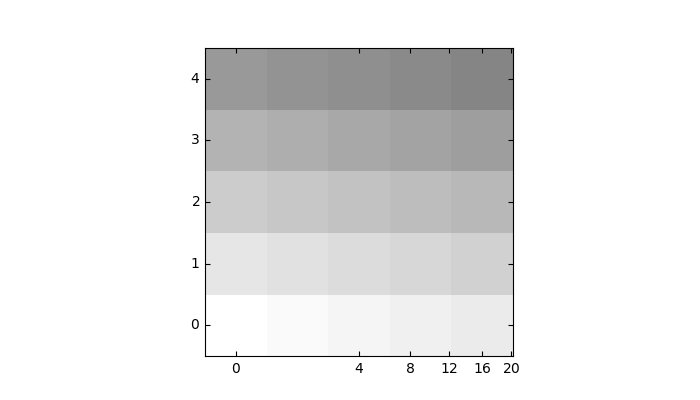# Demo Curvelinear Grid2¶

Custom grid and ticklines.

This example demonstrates how to use GridHelperCurveLinear to define custom grids and ticklines by applying a transformation on the grid. As showcase on the plot, a 5x5 matrix is displayed on the axes.import numpy as np
import matplotlib.pyplot as plt

from mpl_toolkits.axisartist.grid_helper_curvelinear import (
GridHelperCurveLinear)
from mpl_toolkits.axisartist.grid_finder import (
ExtremeFinderSimple, MaxNLocator)
from mpl_toolkits.axisartist.axislines import Subplot

def curvelinear_test1(fig):
"""Grid for custom transform."""

def tr(x, y):
sgn = np.sign(x)
x, y = np.abs(np.asarray(x)), np.asarray(y)
return sgn*x**.5, y

def inv_tr(x, y):
sgn = np.sign(x)
x, y = np.asarray(x), np.asarray(y)
return sgn*x**2, y

grid_helper = GridHelperCurveLinear(
(tr, inv_tr),
extreme_finder=ExtremeFinderSimple(20, 20),
# better tick density
grid_locator1=MaxNLocator(nbins=6), grid_locator2=MaxNLocator(nbins=6))

ax1 = Subplot(fig, 111, grid_helper=grid_helper)
# ax1 will have a ticks and gridlines defined by the given
# transform (+ transData of the Axes). Note that the transform of the Axes
# itself (i.e., transData) is not affected by the given transform.